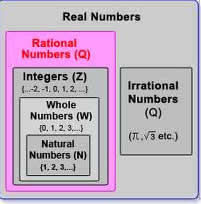# Difference between Real Number and Integer

##### Key Difference: A real number is a number that can take any value on the number line. It can be any of the rational and irrational numbers. Rational number is a number that can be expressed in the form of a fraction but with a non-zero denominator. Integers are types of whole numbers that are not in the form of a fraction. Integers can be both positive and negative.Real numbers consist of all rational as well as irrational numbers. Real numbers can be written in the decimal notation. They include even those that require an infinite decimal expansion. A real number refers to any number that can be found on the number line. The number line can be expressed as an actual geometric line where a point is chosen to be the origin. Points that fall in the right side of origin are considered as positive numbers, whereas numbers lying in the left side of origin are considered to be negative. Therefore, they consist of whole (0, 1 ,3 ,9, 26), rational (6/9, 78.98) and irrational numbers (square root of 3, pi). Infinity does not fall in the category of real numbers. Square root of -1 is also not a real number, and therefore it is referred to as an imaginary number.

Integers are types of whole numbers that are not in the form of fractions. Integers can be both positive and negative. Integers are actually subset of real numbers. It is important to mention that 0 is also included in the list of integers. Zero is considered to be neutral which means that it is neither negative nor positive. Study of integers is primarily done in the theory known as Number theory. Integers are basically used to represent the scenarios where whole numbers cannot be represented in mathematics. For example, bank accounts are associated with both adding and withdrawing of money. Integers are represented on the number line. Every number on the number line has a opposite number, except zero. Therefore, integers also include the opposite numbers in the list.

Comparison between Real Number and Integer:

 Real Number Integer Definition A real number is a number that can take any value on the number line. They can be any of the rational and irrational numbers. Integers can be described as whole numbers meaning that they do not have any fractional parts. Number line Can be plotted on the number line. Can be plotted on the number line. Includes This includes (but is not limited to) positives and negatives, integers and rational numbers, square roots, cube roots , π (pi), etc. Positive integers - they are also know as non-negative integers. (3,5,90) Negative integers - they are opposite of positive integers and have a prefixed sign of ‘-’. (-3, -5, -90) Important points to remember The union of the sets of rational numbers and irrational numbers. Real numbers include zero. Integer numbers include zero. Integers are not in the form of fraction. Origin In the 17th century, Descartes introduced the term "real" to define roots of a polynomial, to separate it from "imaginary" ones. From Latin integer (meaning literally "untouched," hence "whole": the word entire comes from the same origin, but via French.

Image Courtesy: formyschoolstuff.com